# Waveguides Notes | Study Basic Electronics Engineering for SSC JE (Technical) - Electrical Engineering (EE)

## Electrical Engineering (EE): Waveguides Notes | Study Basic Electronics Engineering for SSC JE (Technical) - Electrical Engineering (EE)

The document Waveguides Notes | Study Basic Electronics Engineering for SSC JE (Technical) - Electrical Engineering (EE) is a part of the Electrical Engineering (EE) Course Basic Electronics Engineering for SSC JE (Technical).
All you need of Electrical Engineering (EE) at this link: Electrical Engineering (EE)

Waveguides

Waveguides (single conductor lines) 

• At frequecies higher than 3 GHz, there are more losses in Transmission lines. 
• A hollow metallic tube used to transmit EM waves by successive refelections from inner walls of tube is called waveguide.

Difference with transmission line: 

• In waveguide, only wa ves having frequencies grea ter than cut off frequency fc will be propagated that is why waveguide act as a HPF.
•  Waveguide is one conductor transmission line.

Rectangular waveguide: 

• TM11 is dominant mode for TM waves. 
• TE10 is dominant mode for TE wave and it is also overall dominant mode (when a >b).

In this TM10 or TM01 does not exist.

Cut of frequency :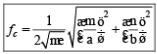Phase constant: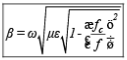Phase velocity: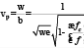Group velocity :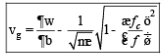In waveguide: vp > c>vg

i.e. vpv=c2

In vacuum : vp = c = vg  Intrinsic wave impedance:

For TE Mode: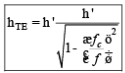For TM mode: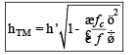Guided wavelength (lg):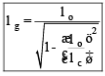Where lo = free space wavelength lc = Cutoff wavelength lg > lc >l0

Power handling capacity of rectangular waveguide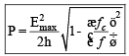OR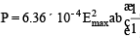For standard rectangular waveguide : a = 2b 

• Dominat mode: it is the mode with lowest cutoff frequency ((longest cutoff wavelength).

Circular /Cylindrical waveguide: 

• TM01 is dominant mode for TM waves. 
• TE11 is dominant node for TE waves and it is overall dominant mode. 
• In circular waveguide second suffix of TE or TM modes cannot be zero (as TMmo or TEmo can not exist)

Cut off wavelength: For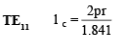r= ra diu s o f c ircula r waveguide

For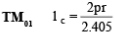Power handling capacity of circular waveguide: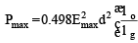d= Intenal diameter
Cavity Frequency: 

• When both the ends of the waveguide are terminated, signal bounces back and forth between two shorting plates. 
• This results in resonance in cavity that is why it is called cavity resonator. 
• Cacity resonators act as BPF and it is used for energy storage.

Resonant frequency: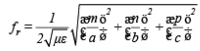• TE101 is the dominant mode of cavity resonator. 
• TM110 is lowest order of cavity resonator.

Quality factor of cavity resonator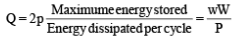W ® Maximum stored energy
P ® average power loss Integer m ® no. of half cycle variations in X direction.
Integer n ® no. of half cycle variations in Y direction.

The document Waveguides Notes | Study Basic Electronics Engineering for SSC JE (Technical) - Electrical Engineering (EE) is a part of the Electrical Engineering (EE) Course Basic Electronics Engineering for SSC JE (Technical).
All you need of Electrical Engineering (EE) at this link: Electrical Engineering (EE)Use Code STAYHOME200 and get INR 200 additional OFF

## Basic Electronics Engineering for SSC JE (Technical)

25 docs|22 tests

Track your progress, build streaks, highlight & save important lessons and more!

,

,

,

,

,

,

,

,

,

,

,

,

,

,

,

,

,

,

,

,

,

;